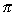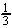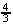Next: Calculator Tips and Programs Up: Study Guide Previous: Integral Calculus

## Some Useful Formulas

loga x =sin2x + cos2x = 1

1 + tan2x = sec2x

1 + cot2x = csc2x

sin 2x = 2 sin x cos x

cos 2x = cos2x - sin2x

sin2x = ½(1- cos 2)

cos2x = ½(1+ cos 2)

Volume of a right circular cylinder =r2h

Volume of a cone =r2h

Volume of a sphere =r3Next: Calculator Tips and Programs Up: Study Guide Previous: Integral Calculus